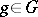# Representation of a group

(diff) ← Older revision | Latest revision (diff) | Newer revision → (diff)
A homomorphism of the group into the group of all invertible transformations of a set. A representationof a groupis called linear ifis a vector space over a fieldand if the transformations,, are linear. Often, linear representations are, for shortness, simply termed representations (cf. Representation theory). In the theory of representations of abstract groups the theory of finite-dimensional representations of finite groups is best developed (cf. Finite group, representation of a; Representation of the symmetric groups).
Ifis a topological group, then one considers continuous linear representations ofon a topological vector space(cf. Continuous representation; Representation of a topological group). Ifis a Lie group andis a finite-dimensional space overor, then a continuous linear representation is automatically real analytic. Analytic and differentiable representations of a Lie group are defined also in the infinite-dimensional case (cf. Analytic representation; Infinite-dimensional representation). To each differentiable representationof a Lie groupcorresponds some linear representation of its Lie algebra — the differential representation of(cf. Representation of a Lie algebra). Ifis moreover connected, then its finite-dimensional representations are completely determined by their differentials. The most developed branch of the representation theory of topological groups is the theory of finite-dimensional linear representations of semi-simple Lie groups, which is often formulated in the language of Lie algebras (cf. Finite-dimensional representation; Representation of the classical groups; Cartan theorem on the highest weight vector), the representation theory of compact groups, and the theory of unitary representations (cf. Representation of a compact group; Unitary representation).